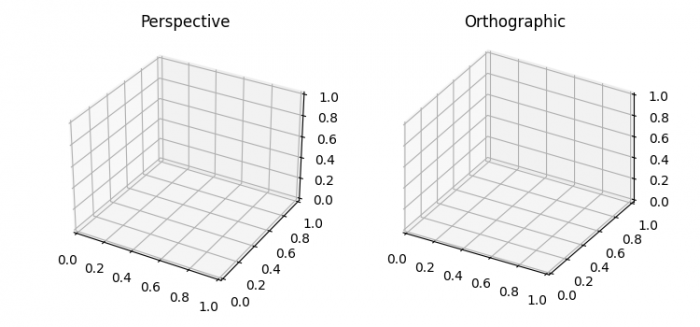# Differentiate the orthographic and perspective projection in Matplotlib

MatplotlibPythonData Visualization

#### Python Data Science basics with Numpy, Pandas and Matplotlib

Most Popular

63 Lectures 6 hours

#### Data Visualization using MatPlotLib & Seaborn

11 Lectures 4 hours

#### MatPlotLib with Python

9 Lectures 2.5 hours

To plot perspective and orthographic projection plots, we can take the following steps −

• Set the figure size and adjust the padding between and around the subplots.
• Create a new figure or activate an existing figure.
• Add an '~.axes.Axes' to the figure as part of a subplot arrangement.
• Set the projection type as 'perspective' on ax1 axis.
• Set the title of the plot.
• Add an '~.axes.Axes' to the figure as part of a subplot arrangement.
• Set the projection type as 'orthographic' on ax2 axis.
• Set the title of the plot.
• To display the figure, use show() method.

## Example

import matplotlib.pyplot as plt
plt.rcParams["figure.figsize"] = [7.50, 3.50]
plt.rcParams["figure.autolayout"] = True

fig = plt.figure()
ax1 = fig.add_subplot(1, 2, 1, projection='3d')
ax1.set_proj_type('persp')
ax1.set_title('Perspective')

ax2 = fig.add_subplot(1, 2, 2, projection='3d')
ax2.set_proj_type('ortho')
ax2.set_title('Orthographic')

plt.show()

## Output